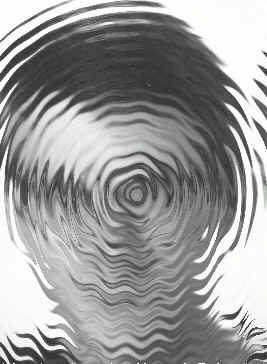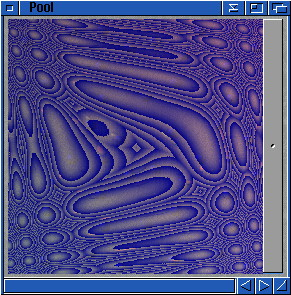This method consists in having a "translation project", which contains how much a pixel has to move in the source project. (Actually it works the other way round, it indicates how far you have to go to fetch the pixel data).
You can then do effects that would either be very difficult or awfully slow to do directely.

With translation masks effects, we will read directely pixels from some source project (zero in the below examples), and get the coordinates where we want to read from another project.

When we are at coordinate (x,y) ( in the destination picture), we will read coordinate (x+dx(x,y) , y+dy(x,y), where dx(x,y) and dy(x,y) are deltas depending on the current (x,y) coordinate. These may be the red and green component of another project, which would mean that, if for instance we want to render coordinate (14,25), we look the mask project (one in the below examples) at coordinate (14,25) (let's say we read the components (10,27,125) there), and add the red/green components to (x,y), so we get dx=10,dy=27, which leads to (24,52). So now we look project zero at coordinate (24,52) and copy the colour to write it into coordinate (14,25) of the destination project...

So, in all these examples, project zero is the source project, one is the mask and two is the destination.

If you want a linear translation, only one component of the mask will be used, and multiplied by different constanty horizontally and vertically:

r(2,x,y)=r(0,x+u*r(1,x,y)/255,y+v*r(1,x,y)/255)
g(2,x,y)=g(0,x+u*r(1,x,y)/255,y+v*r(1,x,y)/255)
b(2,x,y)=b(0,x+u*r(1,x,y)/255,y+v*r(1,x,y)/255)

I let you choose proper values for u and v... The translation will always be done in the direction of vector (u,v). (Of course, I let you replace u/255 by a single value; you may also add a constant to x and y).

Example: In the following picture, I took a picture of a friend, then made the mask using something like sin(sqrt((x-128)^2+(y-128)^2)), and then added something like sin(x)*y, to have the horizontal waves (~~~) in the bottom part of the picture. This is a linear translation mask. (With a (u,v) vector being something like (10,10)...)Two dimensional translation is easy, just read two different components of the mask (here, r and g):

r(2,x,y)=r(0,x+k*r(1,x,y),y+k*g(1,x,y))
g(2,x,y)=g(0,x+k*r(1,x,y),y+k*g(1,x,y))
b(2,x,y)=b(0,x+k*r(1,x,y),y+k*g(1,x,y))

It is possible to load pictures as a mask, pictures you might have done with other paint or picture manipulation programs...

Example: In the following picture (I first created the circled pattern using some MagicWB image and adding a quadratic function I obtained one tile...

I then used an hyperbolic (something like (x-128)/(sqrt((y-128)^2+1)), I do not remember exactely) transformation...Index